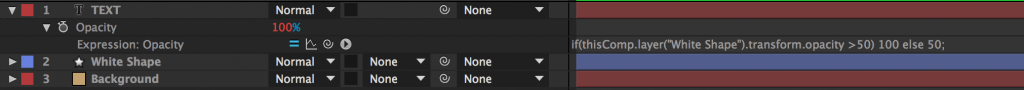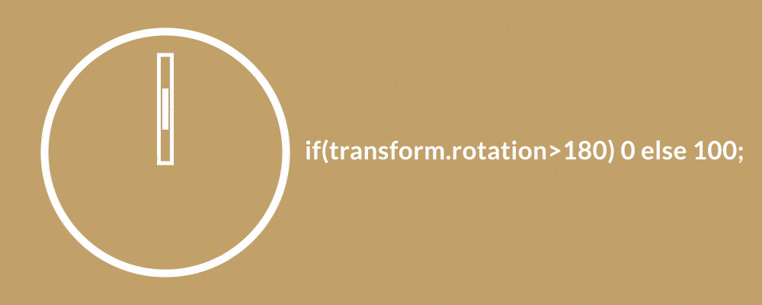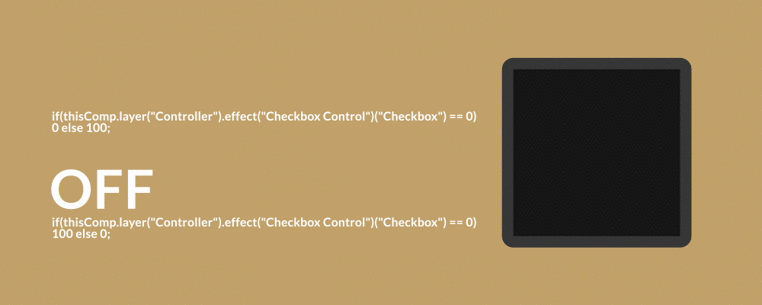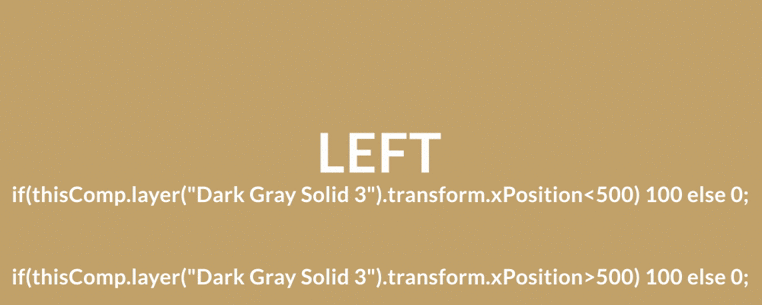Categories

Cover image via

## If/Else expressions are incredibly useful in After Effects…if you know how to use them.

You don’t have to be a computer programmer to get a handle on expressions in After Effects. Let’s dig into the powerful If/Else expression in After Effects.

## What is an If/Else Expression in After Effects?

An If/Else expression in After Effects is a line of code that will change it’s output value based on the input value. If this sounds confusing, don’t worry! It’s simplier than you might think. Let’s take a look at a really basic model:

In this example, the code states that if the white shape’s opacity is more than 50% than the text opacity will be 100%. If the white squares opacity is less than 50% the text opacity will be 50%.Here’s what our After Effects timeline looks like for this example:## How to Write an If/Else Conditional Expression

Writing an If/Else statement is fairly straight forward. Simply open up your expression editor by holding down Option and clicking on the stopwatch next to the parameter you want the If/Else statement to effect. The expression used in the example above is:

if(thisComp.layer(“WhiteShape”).transform.opacity>50) 100 else 50

What do each of the parameters in this expression mean? Let’s  break them down step by step:Write: if(

### 2. Define Your Input Value

Using the pickwhip tool select the value you want to input into your If/Else statement. For the example above, the input value came from the opacity of the white shape. So in that example we would pickwhip to the opacity of the white shape.

### 3. Set Your Equality Operator

An equality operator is essentially a small formula designed to tell your expression what to do. The following chart explains what equality operators you can use in After Effects.

 < Less Than > Greater Than <= Less Than or Equal To >= Greater Than or Equal To == Equals To

For the above example we will use the greater than (>) operator.

Note: There are also more complex operators called “logical operators”. Due to their complexity we will cover those in a later post. However, if you want to learn more check out the If/Else Conditional post at MotionScript.com.

### 4. Define Your Conditional Value

The conditional value can be any number you want. In our example the conditional value was 50.

Write: )

### 6. Add the “true” value

Type in the value you want your expression to output if your conditional statement is true. Look at the example again…if the value of the square was in fact more than 50 (true) than our output value would be 100.

### 7. Define the ‘Else’ Statement

An else statement will define a value if the “if” statement is false. Write: Else

### 8. Add the False value

The false value pertains to the value that will be output if the “if” statement isn’t true. In our example, if the opacity value of the shape was not greater than 50 (false) than the opacity of the text would be 50.

Write: ;

## How Can I Use If/Else Expressions in After Effects?

There are a number of different reasons why a motion graphic designer might want to use If/Else statements in After Effects. Here are just a few.

### Value Based Conditionals

One of the most useful applications of an If/Else statement in After Effects is to have an object’s opacity directly linked to another type of value. For example, the blinking box below has an if statement that says if it’s rotation is greater than 180 than the value should be 0. If the value is less than 180 the value should be 100.### If/Else Conditionals on Checkboxes

Checkboxes are typically used when creating templates for others to use in After Effects. Typically checkboxes control opacity, but they can be used to manipulate other things like color or scale. Checkboxes work with If/Else statements by declaring two values: one if the box is selected (1) and one if the box is not selected (0) using an else statement.

To dive deeper check out our ‘After Effects Quick Tip: Linking Layers to Checkboxes‘ post.### Position Based If/Else Statements

One of the uses best uses that I’ve found for If statements in After Effects is linking layer opacity to an object’s position using an If/Else statement. In short, using this method you can tell certain layers to turn ‘off’ and ‘on’ depending on where an object is currently positioned.### Resources:

If you want to learn more about If/Else statements or expressions in After Effects check out a few of the following resources:

How do you use If/Else statements in After Effects? Share in the comments below.

A